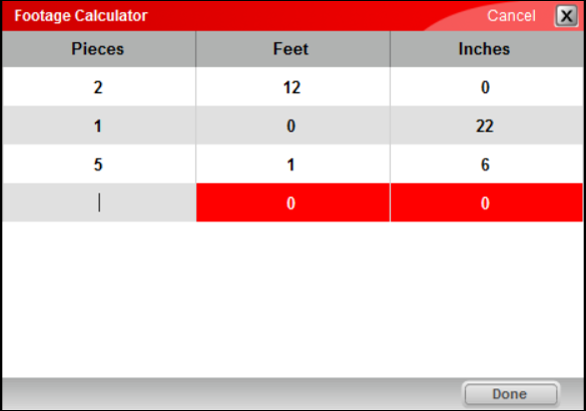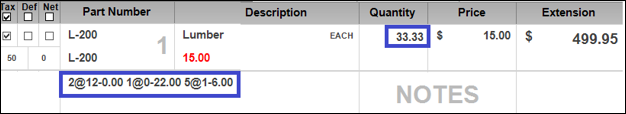## Overview

Paladin provides two calculators for line items that are sold in different lengths or by the square foot.

• The Footage Calculator calculates the line item quantity in feet for one or more pieces of different lengths. Use this calculator for items that you sell by the foot, such as wire and metal roofing.
Example:

• If you enter these lengths and piece quantities:
• 2 piece @  1 foot, 6 inches (1.5 feet)
• 1 piece @ 6 feet
• Then, the line item quantity is set to (2 x 1.5) + (1 x 6) = 9 (feet)
• The Square Footage Calculator calculates the line item quantity in square feet for one or more pieces of length/width dimensions. Use this calculator for items that you sell by the square foot, such as flooring.
Example:

• If you enter these lengths and piece quantities:
• 2 piece @ 1 foot, 6 inches x 2 feet (3 sq ft)
• 1 piece @ 24 inches x 24 inches  (4 sq ft)
• Then, the line item quantity is set to (2 x 3) + (1 x 4 )  =  10 (square feet)

## How to access the Footage Calculator

From the Invoice/Quote module:

1. In Paladin, on the top ribbon, select the Invoice/Quote module.
2. Right-click any line item, then select Footage Calculator.

Alternatives:

• Highlight the invoice item, then press Ctrl + K.
• Highlight the invoice item, then on the Edit menu, click Footage Calculator.

From the PO module:

1. In Paladin, on the top ribbon, select the PO module.
2. Highlight the invoice item, then press Ctrl + K.

Alternative:

• Highlight the invoice item, then on the Edit menu, click Footage Calculator

## How to access the Square Footage Calculator

From the Invoice/Quote module:

1. In Paladin, on the top ribbon, select the Invoice/Quote module.
2. Right-click any line item, then select Square Footage Calculator.

Alternative:

• Highlight the invoice item, then press Ctrl + S.

## How to calculate the total footage (quantity in feet) for invoice items of multiple lengths

The Footage Calculator calculates the line item quantity in feet for one or more pieces of different lengths. Use this calculator for items that you sell by the foot, such as wire and metal roofing.

Once you have opened an item in either Paladin’s Invoice/Quote module or PO module, and have opened the Footage Calculator window, enter the length and number of pieces for each different dimension. Then, click Done.Figure 1: Footage Calculator window

The line item Quantity is set to the total length of all the pieces. A footage summary of values you entered appears as a Notes line item.Figure 2: Footage summary note on invoice

Note: If you need to edit the dimensions or piece quantities, open the Footage Calculator again to make your changes. The line item Quantity will recalculate automatically.

## How to calculate the total square footage (quantity in square feet) for invoice items of multiple dimensions

The Square Footage Calculator calculates the line item quantity in square feet for one or more pieces of different dimensions (length x width). Use this calculator for items that you sell by the square foot, such as flooring.

Once you have opened an item in either Paladin’s Invoice/Quote module or PO module, and have opened the Square Footage Calculator window, for each item with a different dimension enter the number of pieces, the length, and the width. Then, click Done.

The line item  Quantity will be automatically set to the total square footage for all the pieces. A square footage summary shows the data you entered in a separate note line item.Figure 3: Square Footage Calculator window/sq.ft. calculation as note on invoice

Note: If you need to edit the dimensions or piece quantities, open the Square Footage Calculator again and make your changes. The line item Quantity will recalculate automatically.